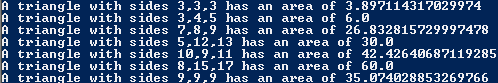# Assignemnt #92, Heron's Formula

## Code

```/// Name: Alex Pinder
/// Period: 5
/// Program Name: Heron's Formula
/// File Name: HeronsFormula.java/HeronsFormulaNoMethod.java
/// Date Complete: 4/15/16

public class HeronsFormula
{
public static void main( String[] args )
{
double a;

a = triangleArea(3, 3, 3);
System.out.println("A triangle with sides 3,3,3 has an area of " + a );

a = triangleArea(3, 4, 5);
System.out.println("A triangle with sides 3,4,5 has an area of " + a );

a = triangleArea(7, 8, 9);
System.out.println("A triangle with sides 7,8,9 has an area of " + a );

System.out.println("A triangle with sides 5,12,13 has an area of " + triangleArea(5, 12, 13) );
System.out.println("A triangle with sides 10,9,11 has an area of " + triangleArea(10, 9, 11) );
System.out.println("A triangle with sides 8,15,17 has an area of " + triangleArea(8, 15, 17) );
System.out.println( "A triangle with sides 9,9,9 has an area of " + triangleArea(9, 9, 9) );
}

public static double triangleArea( double a, double b, double c )
{
// the code in this method computes the area of a triangle whose sides have lengths a, b, and c
double s, A;

s = (a+b+c) / 2.0;
A = Math.sqrt( s*(s-a)*(s-b)*(s-c) );

return A;
// ^ after computing the area, "return" it
}
}
// The output is technically the same for both.
// The line count for the program with methods is 30 while the one without is 50.
// It was much easier to fix the bug in the file with a method because it only requires you to change one value intsead of changing each value individually.
// It was very easy to add the new test becuase I didn't have to write the whole code for finding the area.
```

### Picture of the output